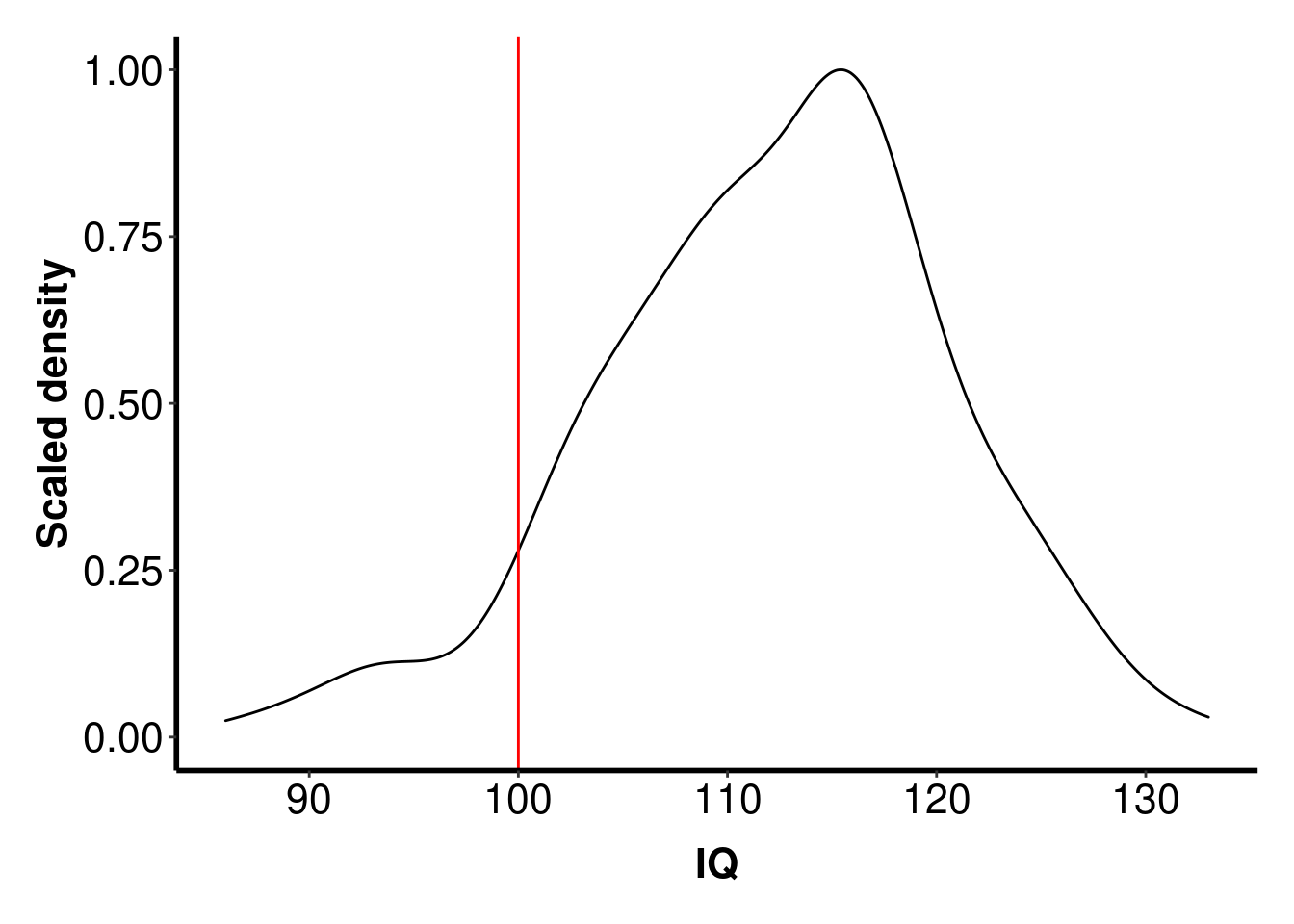# Introduction

In the Absolute Beginners’ Guide to R we used a Bayesian between-subjects t-test to look at the strength of evidence for the presence (or absence) of a difference between groups. Then, in the Intermediate Guide to R, we looked at how to calculate a Bayes Factor for within-subjects experiments. A third, less commonly used, type of t-test is a one-sample t-test, and this is the topic of the current worksheet.

In a one-sample test, you compare the mean of a set of data against a single number; in this worksheet, we’ll compare the mean IQ of a sample of students entering a psychology degree with the known mean IQ of the non-brain damaged population (100).

# Getting started

In the preprocessing worksheet, you loaded data from a git repository into an R project. Go back to that project now. This is very important. Do not stay in your current project, change your Rstudio project using the the drop-down list on the top right of Rstudio. Change to the rminr-data project you used for the preprocessing worksheet. If you do not do this, the rest of this worksheet will not work.

Now that you’re in the rminr-data project, “pull” from the repository to make sure you have the most up to date version. If you’re not sure how to do this, see the data management worksheet.

Finally, create a new script called onesample.R, put all the comments and commands you use in this worksheet into that file, and save regularly.

You will notice that your Environment still contains all the data frames from the previous worksheet. You can leave them there if you like, but some people prefer to start with a “clean slate”. If that’s you, see the within-subjects differences for information on how to clear your environment.

Now load this new data set.

Enter these commands into your script, and run them:

# One-sample Bayesian t-test
library(tidyverse)
iq.data <- read_csv("going-further/iqdata.csv")

Click on the iqdata data frame in the Environment tab, and take a look. You’ll see that its a data frame with 2 columns and 285 rows. The SRN columns contains the student reference number for a student, and the IQ column contains the IQ score for that student.

Note that these data have already been preprocessed - in other words, the students’ performance on individual questions on the IQ test have been combined to give a single score for each participant.

# Exercise: Density plot

Now, as you did in the Absolute Beginners’ Guide to R, WRITE A COMMAND to create a density plot of IQ. Put a red vertical line at the population mean (IQ = 100), change the y-axis label, and add journal styling. Precede your command with an appropriate comment.

If you get it right, it should look like this:If you need some revision, look at previous worksheets on density plots, on drawing vertical lines on graphs, and on making better graphs.

We can see from this plot that the distribution of IQs in our sample peaks higher than the population average of 100. In fact, a rather small proportion of students have an IQ below the population mean.

# Bayesian t-test (one-sample)

We can use the command ttestBF to look at the evidence for this apparent difference between our sample of students and the population mean. We’ve used this command before to do a between-groups test, but we can also use it in a slightly different way to do a one-sample test.

# Load BayesFactor package
library(BayesFactor)
# Conduct one-sample Bayesian t-test

## Explanation of output

The output shows the Bayes Factor, in a similar format to previous Bayes Factor tests you have performed; for details, see the More on Bayes Factors worksheet.

The key figure here is around 1.3 x 1070; recall that this is standard notation and so indicates a very large number. The evidence that the IQs of this group are different (higher) than the population mean of 100 is overwhelming.